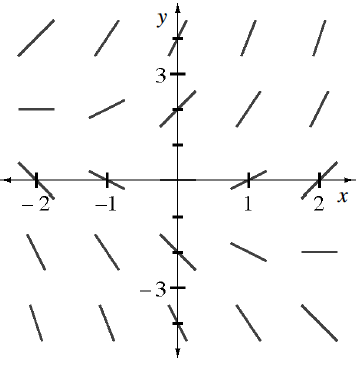### Home > CALC3RD > Chapter Ch9 > Lesson 9.2.2 > Problem9-67

9-67.

Examine the slope field of $\frac { d y } { d x }= x + y$ for $–2 ≤ x ≤ 2$ at right.

1. If $y(-2) = 2$, use Euler’s Method to draw a solution curve for $y$ using $∆x = 1$.

2. Draw a new solution using Euler’s Method if $y(-2) = 0$.Start at $(–2, 2)$. $dy/dx = 0$, so the next point is $(–1, 2)$.

Using $(–1, 2)$, $dy/dx = 1$, so the next point is $(0, 3)$.

Continue this process to locate more points on the solution curve.